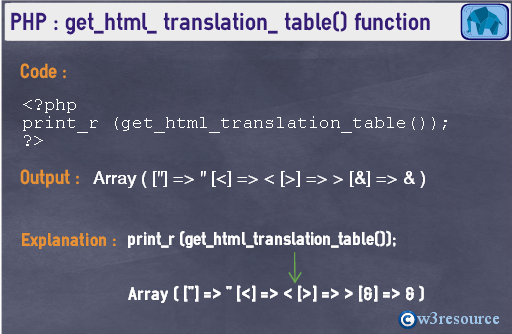# PHP: get_html_ translation_ table() function

## Description

The get_html_translation_table() returns the translation table used by htmlspecialchars() function and htmlentities() function.

Version

(PHP 4 PHP 5 )

Syntax

`get_html_translation_table(table, quote_style)`

Parameters

Name Description Required /
Optional
Type
table For a given string, two constants either HTML_ENTITIES or HTML_SPECIALCHARS, defines what kind of character to html entities mapping is to be done. Optional Integer
quote_style Refers to a style of encoding single and double quote.
ENT_COMPAT : Only convert double quotes, leaving single quotes unaltered. This is the default one.
ENT_QUOTES : Converts both single and double quotes.
ENT_NOQUOTES: Converts neither single nor double quotes.
Optional Integer

Return values:

The translation table as an array.

Value Type: Array.

Pictorial PresentationExample:

``<?phpprint_r (get_html_translation_table());echo '<br>';print_r (get_html_translation_table(HTML_ENTITIES));echo '<br>';print_r (get_html_translation_table(HTML_SPECIALCHARS,ENT_QUOTES));?>``

Output:

`Array  (      ["] => "      [<] => <      [>] => >      [&] => &  ) Array  (      [ ] =>        [¡] => ¡      [¢] => ¢      [£] => £      [¤] => ¤      [¥] => ¥      [¦] => ¦      [§] => §      [¨] => ¨      [©] => ©      [ª] => ª      [«] => «      [¬] => ¬      [­] => ­      [®] => ®      [¯] => ¯      [°] => °      [±] => ±      [²] => ²      [³] => ³      [´] => ´      [µ] => µ      [¶] => ¶      [·] => ·      [¸] => ¸      [¹] => ¹      [º] => º      [»] => »      [¼] => ¼      [½] => ½      [¾] => ¾      [¿] => ¿      [À] => À      [Á] => Á      [Â] => Â      [Ã] => Ã      [Ä] => Ä      [Å] => Å      [Æ] => Æ      [Ç] => Ç      [È] => È      [É] => É      [Ê] => Ê      [Ë] => Ë      [Ì] => Ì      [Í] => Í      [Î] => Î      [Ï] => Ï      [Ð] => Ð      [Ñ] => Ñ      [Ò] => Ò      [Ó] => Ó      [Ô] => Ô      [Õ] => Õ      [Ö] => Ö      [×] => ×      [Ø] => Ø      [Ù] => Ù      [Ú] => Ú      [Û] => Û      [Ü] => Ü      [Ý] => Ý      [Þ] => Þ      [ß] => ß      [à] => à      [á] => á      [â] => â      [ã] => ã      [ä] => ä      [å] => å      [æ] => æ      [ç] => ç      [è] => è      [é] => é      [ê] => ê      [ë] => ë      [ì] => ì      [í] => í      [î] => î      [ï] => ï      [ð] => ð      [ñ] => ñ      [ò] => ò      [ó] => ó      [ô] => ô      [õ] => õ      [ö] => ö      [÷] => ÷      [ø] => ø      [ù] => ù      [ú] => ú      [û] => û      [ü] => ü      [ý] => ý      [þ] => þ      [ÿ] => ÿ      ["] => "      [<] => <      [>] => >      [&] => &  ) Array  (      ["] => "      ['] => '      [<] => <      [>] => >      [&] => &  ) `

View the example in the browser

PHP Function Reference

Previous: fprintf
Next: hebrev

﻿

## PHP: Tips of the Day

In PHP, there are two versions of logical AND and OR operators.

Operator True if
\$a and \$b Both \$a and \$b are true
\$a && \$b Both \$a and \$b are true
\$a or \$b Either \$a or \$b is true
\$a || \$b Either \$a or \$b is true

Note that the && and || opererators have higher precedence than and and or. See table below:

Evaluation Result of \$e Evaluated as
\$e = false || true True \$e = (false || true)
\$e = false or true False (\$e = false) or true

Because of this it's safer to use && and || instead of and and or.